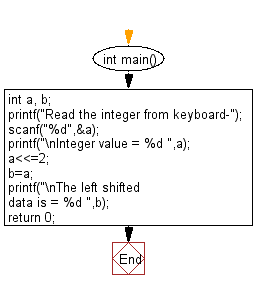﻿ C Program: Shift the specified data by two bits to the left - w3resource

# C Exercises: Shift the specified data by two bits to the left

## C Basic Declarations and Expressions: Exercise-56 with Solution

Write a C program to shift given data by two bits to the left.

Sample Solution:

C Code:

``````#include<stdio.h>
int main() {
int a, b;

// Prompt user to input an integer
scanf("%d",&a);

// Display the original integer value
printf("\nInteger value = %d ",a);

// Left shift 'a' by 2 bits and assign it to 'b'
a <<= 2;
b = a;

// Display the left shifted data
printf("\nThe left shifted data is = %d ",b);

return 0;
}
``````

Sample Output:

```Read the integer from keyboard-
Integer value = 2
The left shifted data is = 8
```

Flowchart:C programming Code Editor:

What is the difficulty level of this exercise?

Test your Programming skills with w3resource's quiz.

﻿